## 1 Introduction

Many theorem provers have the ability to generate executable code in some (typically functional) programming language from definitions, lemmas and proofs (e.g. [6, 8, 9, 12, 16, 27, 37]). This makes code generation part of the trusted kernel of the system. Myreen and Owens  closed this gap for the HOL4 system: they have implemented a tool that translates from HOL4 into CakeML, a subset of SML, and proves a theorem stating that a result produced by the CakeML code is correct w.r.t. the HOL functions. They also have a verified implementation of CakeML [24, 40]. We go one step further and provide a once-and-for-all verified compiler from (deeply embedded) function definitions in Isabelle/HOL [32, 33] into CakeML proving partial correctness of the generated CakeML code w.r.t. the original functions. This is like the step from dynamic to static type checking. It also means that preconditions on the input to the compiler are explicitly given in the correctness theorem rather than implicitly by a failing translation. To the best of our knowledge this is the first verified (as opposed to certifying) compiler from function definitions in a logic into a programming language.

Our compiler is composed of multiple phases and in principle applicable to other languages than Isabelle/HOL or even HOL:

• We erase types right away. Hence the type system of the source language is irrelevant.

• We merely assume that the source language has a semantics based on equational logic.

The compiler operates in three stages:

1. 1.

The preprocessing phase eliminates features that are not supported by our compiler. Most importantly, dictionary construction eliminates occurrences of type classes in HOL terms. It introduces dictionary datatypes and new constants and proves the equivalence of old and new constants (Sect. 7).

2. 2.

The deep embedding lifts HOL terms into terms of type $$\mathsf {term}$$, a HOL model of HOL terms. For each constant c (of arbitrary type) it defines a constant $$c'$$ of type $$\mathsf {term}$$ and proves a theorem that expresses equivalence (Sect. 3).

3. 3.

There are multiple compiler phases that eliminate certain constructs from the $$\mathsf {term}$$ type, until we arrive at the CakeML expression type. Most phases target a different intermediate term type (Sect. 5).

The first two stages are preprocessing, are implemented in ML and produce certificate theorems. Only these stages are specific to Isabelle. The third (and main) stage is implemented completely in the logic HOL, without recourse to ML. Its correctness is verified once and for all.Footnote 1

## 2 Related Work

There is existing work in the Coq [2, 15] and HOL  communities for proof producing or verified extraction of functions defined in the logic. Anand et al.  present work in progress on a verified compiler from Gallina (Coq’s specification language) via untyped intermediate languages to CompCert C light. They plan to connect their extraction routine to the CompCert compiler .

Translation of type classes into dictionaries is an important feature of Haskell compilers. In the setting of Isabelle/HOL, this has been described by Wenzel  and Krauss et al. . Haftmann and Nipkow  use this construction to compile HOL definitions into target languages that do not support type classes, e.g. Standard ML and OCaml. In this work, we provide a certifying translation that eliminates type classes inside the logic.

Compilation of pattern matching is well understood in literature [3, 36, 38]. In this work, we contribute a transformation of sets of equations with pattern matching on the left-hand side into a single equation with nested pattern matching on the right-hand side. This is implemented and verified inside Isabelle.

Besides CakeML, there are many projects for verified compilers for functional programming languages of various degrees of sophistication and realism (e.g. [4, 11, 14]). Particularly modular is the work by Neis et al.  on a verified compiler for an ML-like imperative source language. The main distinguishing feature of our work is that we start from a set of higher-order recursion equations with pattern matching on the left-hand side rather than a lambda calculus with pattern matching on the right-hand side. On the other hand we stand on the shoulders of CakeML which allows us to bypass all complications of machine code generation. Note that much of our compiler is not specific to CakeML and that it would be possible to retarget it to, for example, Pilsner abstract syntax with moderate effort.

Finally, Fallenstein and Kumar  have presented a model of HOL inside HOL using large cardinals, including a reflection proof principle.

## 3 Deep Embedding

Starting with a HOL definition, we derive a new, reified definition in a deeply embedded term language depicted in Fig. 1a. This term language corresponds closely to the term datatype of Isabelle’s implementation (using de Bruijn indices ), but without types and schematic variables.

To establish a formal connection between the original and the reified definitions, we use a logical relation, a concept that is well-understood in literature  and can be nicely implemented in Isabelle using type classes. Note that the use of type classes here is restricted to correctness proofs; it is not required for the execution of the compiler itself. That way, there is no contradiction to the elimination of type classes occurring in a previous stage.

Notation. We abbreviate $$\mathsf {App}\;t\;u$$ to t \$ u and $$\mathsf {Abs}\;t$$ to $$\varLambda \;t$$. Other term types introduced later in this paper use the same conventions. We reserve $$\lambda$$ for abstractions in HOL itself. Typing judgments are written with a double colon: $$t\, {:}{:}\, \tau$$.

Embedding Operation. Embedding is implemented in ML. We denote this operation using angle brackets: $$\left\langle t\right\rangle$$, where t is an arbitrary HOL expression and the result $$\left\langle t\right\rangle$$ is a HOL value of type $$\mathsf {term}$$. It is a purely syntactic transformation, without preliminary evaluation or reduction, and it discards type information. The following examples illustrate this operation and typographical conventions concerning variables and constants:

Small-Step Semantics. Figure 1b specifies the small-step semantics for $$\mathsf {term}$$. It is reminiscent of higher-order term rewriting, and modelled closely after equality in HOL. The basic idea is that if the proposition $$t = u$$ can be proved equationally in HOL (without symmetry), then $$R \vdash {\left\langle t\right\rangle } \longrightarrow ^* {\left\langle u\right\rangle }$$ holds (where $$\textit{R}\, {:}{:}\, (\mathsf {term} \times \mathsf {term})\;\mathsf {set}$$). We call $$\textit{R}$$ the rule set. It is the result of translating a set of defining equations $$lhs = rhs$$ into pairs $$(\left\langle lhs \right\rangle , \left\langle rhs \right\rangle ) \in \textit{R}$$.

Rule Step performs a rewrite step by picking a rewrite rule from R and rewriting the term at the root. For that purpose, $$\mathsf {match}$$ and $$\mathsf {subst}$$ are (mostly) standard first-order matching and substitution (see Sect. 4 for details).

Rule Beta performs $$\beta$$-reduction. Type $$\mathsf {term}$$ represents bound variables by de Bruijn indices. The notation $$t [t']$$ represents the substitution of the outermost bound variable in t with $$t'$$.

Our semantics does not constitute a fully-general higher-order term rewriting system, because we do not allow substitution under binders. For de Bruijn terms, this would pose no problem, but as soon as we introduce named bound variables, substitution under binders requires dealing with capture. To avoid this altogether, all our semantics expect terms that are substituted into abstractions to be closed. However, this does not mean that we restrict ourselves to any particular evaluation order. Both call-by-value and call-by-name can be used in the small-step semantics. But later on, the target semantics will only use call-by-value.

Embedding Relation. We denote the concept that an embedded term t corresponds to a HOL term a of type $$\tau$$ w.r.t. rule set $$\textit{R}$$ with the syntax $$\textit{R} \vdash t \approx a$$. If we want to be explicit about the type, we index the relation: $$\approx _\tau$$.

For ground types, this can be defined easily. For example, the following two rules define $$\approx _{\mathsf {nat}}$$: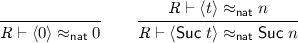Definitions of $$\approx$$ for arbitrary datatypes without nested recursion can be derived mechanically in the same fashion as for $$\mathsf {nat}$$, where they constitute one-to-one relations. Note that for ground types, $$\approx$$ ignores $$\textit{R}$$. The reason why $$\approx$$ is parametrized on $$\textit{R}$$ will become clear in a moment.

For function types, we follow Myreen and Owen’s approach . The statement $$\textit{R} \vdash t \approx f$$ can be interpreted as “$$t\mathbin {\}\left\langle a\right\rangle$$ can be rewritten to $$\left\langle f\;a\right\rangle$$ for all a”. Because this might involve applying a function definition from $$\textit{R}$$, the $$\approx$$ relation must be indexed by the rule set. As a notational convenience, we define another relation $$\textit{R} \vdash t \downarrow x$$ to mean that there is a $$t'$$ such that $$R \vdash {t} \longrightarrow ^* {t'}$$ and $$\textit{R} \vdash t' \approx x$$. Using this notation, we formally define $$\approx$$ for functions as follows:

$$\textit{R} \vdash t \approx f \leftrightarrow (\forall u \; x.\; \textit{R} \vdash u \downarrow x \rightarrow \textit{R} \vdash t\mathbin {\}u \downarrow f\;x)$$

Example. As a running example, we will use the $$\mathsf {map}$$ function on lists:The result of embedding this function is a set of rules $$\mathsf {map'}$$:

The induction principle for the proof arises from the use of the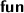command that is used to define recursive functions in HOL . But the user is also allowed to specify custom equations for functions, in which case we will use heuristics to generate and prove the appropriate induction theorem. For simplicity, we will use the term (defining) equation uniformly to refer to any set of equations, either default ones or ones specified by the user. Embedding partially-specified functions – in particular, proving the certificate theorem about them – is currently not supported. In the future, we plan to leverage the domain predicate as produced by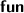to generate conditional theorems.

## 4 Terms, Matching and Substitution

The compiler transforms the initial $$\mathsf {term}$$ type (Fig. 1a) through various intermediate stages. This section gives an overview and introduces necessary terminology.

Preliminaries. The function arrow in HOL is $$\Rightarrow$$. The cons operator on lists is the infix $$\#$$.

Throughout the paper, the concept of mappings is pervasive: We use the type notation $$\alpha \rightharpoonup \beta$$ to denote a function $$\alpha \Rightarrow \beta \;\mathsf {option}$$. In certain contexts, a mapping may also be called an environment. We write mapping literals using brackets: $$[a \Rightarrow x, b \Rightarrow y, \ldots ]$$. If it is clear from the context that $$\sigma$$ is defined on a, we often treat the lookup $$\sigma \;a$$ as returning an $$x\, {:}{:}\, \beta$$.

The functions $$\mathsf {dom}\, {:}{:}\, (\alpha \rightharpoonup \beta ) \Rightarrow \alpha \;\mathsf {set}$$ and $$\mathsf {range}\, {:}{:}\, (\alpha \rightharpoonup \beta ) \Rightarrow \beta \;\mathsf {set}$$ return the domain and range of a mapping, respectively.

Dropping entries from a mapping is denoted by $$\sigma - k$$, where $$\sigma$$ is a mapping and k is either a single key or a set of keys. We use $$\sigma ' \subseteq \sigma$$ to denote that $$\sigma '$$ is a sub-mapping of $$\sigma$$, that is, $$\mathsf {dom}\;\sigma ' \subseteq \mathsf {dom}\;\sigma$$ and $$\forall a \in \mathsf {dom}\;\sigma '.\; \sigma '\;a = \sigma \;a$$.

Merging two mappings $$\sigma$$ and $$\rho$$ is denoted with $$\sigma \mathbin {+\!\!+}\rho$$. It constructs a new mapping with the union domain of $$\sigma$$ and $$\rho$$. Entries from $$\rho$$ override entries from $$\sigma$$. That is, $$\rho \subseteq \sigma \mathbin {+\!\!+}\rho$$ holds, but not necessarily $$\sigma \subseteq \sigma \mathbin {+\!\!+}\rho$$.

All mappings and sets are assumed to be finite. In the formalization, this is enforced by using subtypes of $$\rightharpoonup$$ and $$\mathsf {set}$$. Note that one cannot define datatypes by recursion through sets for cardinality reasons. However, for finite sets, it is possible. This is required to construct the various term types. We leverage facilities of Blanchette et al.’s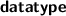command to define these subtypes .

Standard Functions. All type constructors that we use ($$\rightharpoonup$$, $$\mathsf {set}$$, $$\mathsf {list}$$, $$\mathsf {option}$$, ...) support the standard operations $$\mathsf {map}$$ and $$\mathsf {rel}$$. For lists, $$\mathsf {map}$$ is the regular covariant map. For mappings, the function has the type $$(\beta \Rightarrow \gamma ) \Rightarrow (\alpha \rightharpoonup \beta ) \Rightarrow (\alpha \rightharpoonup \gamma )$$. It leaves the domain unchanged, but applies a function to the range of the mapping.

Function $$\mathsf {rel}_\tau$$ lifts a binary predicate $$P\, {:}{:}\, \alpha \Rightarrow \alpha \Rightarrow \mathsf {bool}$$ to the type constructor $$\tau$$. We call this lifted relation the relator for a particular type.

For datatypes, its definition is structural, for example: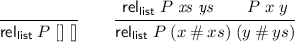For sets and mappings, the definition is a little bit more subtle.

### Definition 1 (Set relator)

For each element $$a \in A$$, there must be a corresponding element $$b \in B$$ such that $$P\;a\;b$$, and vice versa. Formally:

\begin{aligned} \mathsf {rel}_\mathsf {set}\;P\;A\;B \leftrightarrow (\forall x \in A.\; \exists y \in B.\; P\;x\;y) \wedge (\forall y \in B.\; \exists x \in A.\; P\;x\;y) \end{aligned}

### Definition 2 (Mapping relator)

For each a, $$m\;a$$ and $$n\;a$$ must be related according to $$\mathsf {rel}_\mathsf {option}\;P$$. Formally:

\begin{aligned} \mathsf {rel}_\mathsf {mapping}\;P\;m\;n \leftrightarrow (\forall a.\; \mathsf {rel}_\mathsf {option}\;P\;(m\;a)\;(n\;a)) \end{aligned}

Term Types. There are four distinct term types: $$\mathsf {term}$$, $$\mathsf {nterm}$$, $$\mathsf {pterm}$$, and $$\mathsf {sterm}$$. All of them support the notions of free variables, matching and substitution. Free variables are always a finite set of strings. Matching a term against a pattern yields an optional mapping of type $$\mathsf {string} \rightharpoonup \alpha$$ from free variable names to terms.

Note that the type of patterns is itself $$\mathsf {term}$$ instead of a dedicated pattern type. The reason is that we have to subject patterns to a linearity constraint anyway and may use this constraint to carve out the relevant subset of terms:

### Definition 3

A term is linear if there is at most one occurrence of any variable, it contains no abstractions, and in an application $$f\mathbin {\}x$$, f must not be a free variable. The HOL predicate is called $$\mathsf {linear}\, {:}{:}\, \mathsf {term} \Rightarrow \mathsf {bool}$$.

Because of the similarity of operations across the term types, they are all instances of the $$\mathsf {term}$$ type class. Note that in Isabelle, classes and types live in different namespaces. The $$\mathsf {term}$$ type and the $$\mathsf {term}$$ type class are separate entities.

### Definition 4

A term type $$\tau$$ supports the operations $$\mathsf {match}\, {:}{:}\, \mathsf {term} \Rightarrow \tau \Rightarrow (\mathsf {string} \rightharpoonup \tau )$$, $$\mathsf {subst}\, {:}{:}\, (\mathsf {string} \rightharpoonup \tau ) \Rightarrow \tau \Rightarrow \tau$$ and $$\mathsf {frees}\, {:}{:}\, \tau \Rightarrow \mathsf {string}\;\mathsf {set}$$. We also define the following derived functions:

• $$\mathsf {matchs}$$ matches a list of patterns and terms sequentially, producing a single mapping

• $$\mathsf {closed}\;t$$ is an abbreviation for $$\mathsf {frees}\;t = \emptyset$$

• $$\mathsf {closed}\;\sigma$$ is an overloading of $$\mathsf {closed}$$, denoting that all values in a mapping are closed

Additionally, some (obvious) axioms have to be satisfied. We do not strive to fully specify an abstract term algebra. Instead, the axioms are chosen according to the needs of this formalization.

A notable deviation from matching as discussed in term rewriting literature is that the result of matching is only well-defined if the pattern is linear.

### Definition 5

An equation is a pair of a pattern (left-hand side) and a term (right-hand side). The pattern is of the form $$f\mathbin \p_1\mathbin \\ldots \mathbin \p_n$$, where f is a constant (i.e. of the form $$\mathsf {Const}\; name$$). We refer to both f or $$name$$ interchangeably as the function symbol of the equation.

Following term rewriting terminology, we sometimes refer to an equation as rule.

### 4.1 De Bruijn terms ()

The definition of $$\mathsf {term}$$ is almost an exact copy of Isabelle’s internal term type, with the notable omissions of type information and schematic variables (Fig. 1a). The implementation of $$\beta$$-reduction is straightforward via index shifting of bound variables.

### 4.2 Named Bound Variables (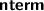)

The $$\mathsf {nterm}$$ type is similar to $$\mathsf {term}$$, but removes the distinction between bound and free variables. Instead, there are only named variables. As mentioned in the previous section, we forbid substitution of terms that are not closed in order to avoid capture. This is also reflected in the syntactic side conditions of the correctness proofs (Sect. 5.1).

### 4.3 Explicit Pattern Matching (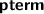)

Functions in HOL are usually defined using implicit pattern matching, that is, the terms $$p_i$$ occurring on the left-hand side $$\left\langle \mathsf {f}\;p_1\;\ldots \;p_n\right\rangle$$ of an equation must be constructor patterns. This is also common among functional programming languages like Haskell or OCaml. CakeML only supports explicit pattern matching using case expressions. A function definition consisting of multiple defining equations must hence be translated to the form $$f = \lambda x.\;\mathsf {\mathbf {case}}\;x\;\mathsf {\mathbf {of}}\;\ldots$$. The elimination proceeds by iteratively removing the last parameter in the block of equations until none are left.

In our formalization, we opted to combine the notion of abstraction and case expression, yielding case abstractions, represented as the $$\mathsf {Pabs}$$ constructor. This is similar to the fn construct in Standard ML, which denotes an anonymous function that immediately matches on its argument . The same construct also exists in Haskell with the LambdaCase language extension. We chose this representation mainly for two reasons: First, it allows for a simpler language grammar because there is only one (shared) constructor for abstraction and case expression. Second, the elimination procedure outlined above does not have to introduce fresh names in the process. Later, when translating to CakeML syntax, fresh names are introduced and proved correct in a separate step.

The set of pairs of pattern and right-hand side inside a case abstraction is referred to as clauses. As a short-hand notation, we use $$\varLambda \{ p_1 \Rightarrow t_1, p_2 \Rightarrow t_2, \ldots \}$$.

### 4.4 Sequential Clauses (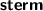)

In the term rewriting fragment of HOL, the order of rules is not significant. If a rule matches, it can be applied, regardless when it was defined or proven. This is reflected by the use of sets in the rule and term types. For CakeML, the rules need to be applied in a deterministic order, i.e. sequentially. The $$\mathsf {sterm}$$ type only differs from $$\mathsf {pterm}$$ by using $$\mathsf {list}$$ instead of $$\mathsf {set}$$. Hence, case abstractions use list brackets: $$\varLambda [p_1 \Rightarrow t_1, p_2 \Rightarrow t_2, \ldots ]$$.

### 4.5 Irreducible Terms (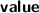)

CakeML distinguishes between expressions and values. Whereas expressions may contain free variables or $$\beta$$-redexes, values are closed and fully evaluated. Both have a notion of abstraction, but values differ from expressions in that they contain an environment binding free variables.

Consider the expression $$(\lambda x. \lambda y. x)\,(\lambda z. z)$$, which is rewritten (by $$\beta$$-reduction) to $$\lambda y. \lambda z. z$$. Note how the bound variable x disappears, since it is replaced. This is contrary to how programming languages are usually implemented: evaluation does not happen by substituting the argument term t for the bound variable x, but by recording the binding $$x \mapsto t$$ in an environment . A pair of an abstraction and an environment is usually called a closure [25, 41].

In CakeML, this means that evaluation of the above expression results in the closure

Note the nested structure of the closure, whose environment itself contains a closure.

To reflect this in our formalization, we introduce a type $$\mathsf {value}$$ of values (explanation inline):

The above example evaluates to the closure:

The third case for recursive closures only becomes relevant when we conflate variables and constants. As long as the rule set $$\textit{rs}$$ is kept separate, recursive calls are straightforward: the appropriate definition for the constant can be looked up there. CakeML knows no such distinction between constants and variables, hence everything has to reside in a single environment $$\sigma$$.

Consider this example of $$\mathsf {odd}$$ and $$\mathsf {even}$$:

\begin{aligned} \mathsf {odd}\;0&= \mathsf {False}&\mathsf {even}\;0&= \mathsf {True}\\ \mathsf {odd}\;(\mathsf {Suc}\;n)&= \mathsf {even}\;n&\mathsf {even}\;(\mathsf {Suc}\;n)&= \mathsf {odd}\;n \end{aligned}

When evaluating the term $$\mathsf {odd}\;k$$, the definitions of $$\mathsf {even}$$ and $$\mathsf {odd}$$ themselves must be available in the environment captured in the definition of $$\mathsf {odd}$$. However, it would be cumbersome in HOL to construct such a $$\mathsf {Vabs}$$ that refers to itself. Instead, we capture the expressions used to define $$\mathsf {odd}$$ and $$\mathsf {even}$$ in a recursive closure. Other encodings might be possible, but since we are targeting CakeML, we are opting to model it in a similar way as its authors do.

For the above example, this would result in the following global environment:

Note that in the first line, the right-hand sides are values, but in $$\textit{css}$$, they are expressions. The additional $$\mathsf {string}$$ argument of $$\mathsf {Vrecabs}$$ denotes the selected function. When evaluating an application of a recursive closure to an argument ($$\beta$$-reduction), the semantics adds all constituent functions of the closure to the environment used for recursive evaluation.

## 5 Intermediate Semantics and Compiler Phases

In this section, we will discuss the progression from de Bruijn based term language with its small-step semantics given in Fig. 1a to the final CakeML semantics. The compiler starts out with terms of type $$\mathsf {term}$$ and applies multiple phases to eliminate features that are not present in the CakeML source language. Types $$\mathsf {term}$$, $$\mathsf {nterm}$$ and $$\mathsf {pterm}$$ each have a small-step semantics only. Type $$\mathsf {sterm}$$ has a small-step and several intermediate big-step semantics that bridge the gap to CakeML. An overview of the intermediate semantics and compiler phases is depicted in Fig. 2. The left-hand column gives an overview of the different phases. The right-hand column gives the types of the rule set and the semantics for each phase; you may want to skip it upon first reading.

### 5.1 Side Conditions

All of the following semantics require some side conditions on the rule set. These conditions are purely syntactic. As an example we list the conditions for the correctness of the first compiler phase:

• Patterns must be linear, and constructors in patterns must be fully applied.

• Definitions must have at least one parameter on the left-hand side (Sect. 5.6).

• The right-hand side of an equation refers only to free variables occurring in patterns on the left-hand side and contain no dangling de Bruijn indices.

• There are no two defining equations $$lhs = rhs _1$$ and $$lhs = rhs _2$$ such that $$rhs _1 \ne rhs _2$$.

• For each pair of equations that define the same constant, their arity must be equal and their patterns must be compatible (Sect. 5.3).

• There is at least one equation.

• Variable names occurring in patterns must not overlap with constant names (Sect. 5.7).

• Any occurring constants must either be defined by an equation or be a constructor.

The conditions for the subsequent phases are sufficiently similar that we do not list them again.

In the formalization, we use named contexts to fix the rules and assumptions on them (locales in Isabelle terminology). Each phase has its own locale, together with a proof that after compilation, the preconditions of the next phase are satisfied. Correctness proofs assume the above conditions on R and similar conditions on the term that is reduced. For brevity, this is usually omitted in our presentation.

### 5.2 Naming Bound Variables: From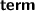toIsabelle uses de Bruijn indices in the term language for the following two reasons: For substitution, there is no need to rename bound variables. Additionally, $$\alpha$$-equivalent terms are equal. In implementations of programming languages, these advantages are not required: Typically, substitutions do not happen inside abstractions, and there is no notion of equality of functions. Therefore CakeML uses named variables and in this compilation step, we get rid of de Bruijn indices.

The “named” semantics is based on the $$\mathsf {nterm}$$ type. The rules that are changed from the original semantics (Fig. 1b) are given in Fig. 3 (Fun and Arg remain unchanged). Notably, $$\beta$$-reduction reuses the substitution function.

For the correctness proof, we need to establish a correspondence between $$\mathsf {term}$$s and $$\mathsf {nterm}$$s. Translation from $$\mathsf {nterm}$$ to $$\mathsf {term}$$ is trivial: Replace bound variables by the number of abstractions between occurrence and where they were bound in, and keep free variables as they are. This function is called $$\mathsf {nterm\_to\_term}$$.

The other direction is not unique and requires introduction of fresh names for bound variables. In our formalization, we have chosen to use a monad to produce these names. This function is called $$\mathsf {term\_to\_nterm}$$. We can also prove the obvious property $$\mathsf {nterm\_to\_term}\;(\mathsf {term\_to\_nterm}\;t) = t$$, where t is a $$\mathsf {term}$$ without dangling de Bruijn indices.

Generation of fresh names in general can be thought of as picking a string that is not an element of a (finite) set of already existing names. For Isabelle, the Nominal framework [42, 43] provides support for reasoning over fresh names, but unfortunately, its definitions are not executable.

Instead, we chose to model generation of fresh names as a monad $$\alpha \;\mathsf {fresh}$$ with the following primitive operations in addition to the monad operations:

\begin{aligned} \mathsf {run}&{:}{:}\,\, \alpha \;\mathsf {fresh} \Rightarrow \mathsf {string}\;\mathsf {set} \Rightarrow \alpha \\ \mathsf {fresh\_name}&{:}{:}\,\, \mathsf {string}\;\mathsf {fresh} \end{aligned}

In our implementation, we have chosen to represent $$\alpha \;\mathsf {fresh}$$ as roughly isomorphic to the state monad.

Compilation of a rule set proceeds by translation of the right-hand side of all rules:

$$\mathsf {compile}\;\textit{R}= \{ (p, \mathsf {term\_to\_nterm}\;t) \;|\; (p, t) \in \textit{R}\}$$

The left-hand side is left unchanged for two reasons: function $$\mathsf {match}$$ expects an argument of type $$\mathsf {term}$$ (see Sect. 4), and patterns do not contain abstractions or bound variables.

### Theorem 1 (Correctness of compilation)

Assuming a step can be taken with the compiled rule set, it can be reproduced with the original rule set.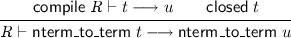We prove this by induction over the semantics (Fig. 3).

### 5.3 Explicit Pattern Matching: Fromto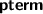Usually, functions in HOL are defined using implicit pattern matching, that is, the left-hand side of an equation is of the form $$\left\langle \mathsf {f}\;p_1\;\ldots \;p_n\right\rangle$$, where the $$p_i$$ are patterns over datatype constructors. For any given function $$\mathsf {f}$$, there may be multiple such equations. In this compilation step, we transform sets of equations for $$\mathsf {f}$$ defined using implicit pattern matching into a single equation for $$\mathsf {f}$$ of the form $$\left\langle \mathsf {f}\right\rangle = \varLambda \;\textit{C}$$, where $$\textit{C}$$ is a set of clauses.

The strategy we employ currently requires successive elimination of a single parameter from right to left, in a similar fashion as Slind’s pattern matching compiler [38, Sect. 3.3.1]. Recall our running example ($$\mathsf {map}$$). It has arity 2. We omit the brackets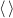for brevity. First, the list parameter gets eliminated: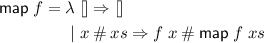Finally, the function parameter gets eliminated:

\begin{aligned} \mathsf {map} = \lambda \; f \Rightarrow \big (\lambda \;&[] \Rightarrow [] \\ |\;&x \mathbin {\#}xs \Rightarrow f\;x \mathbin {\#}\mathsf {map}\;f\;xs\big ) \end{aligned}

This has now arity 0 and is defined by a twice-nested abstraction.

Semantics. The target semantics is given in Fig. 4 (the Fun and Arg rules from previous semantics remain unchanged). We start out with a rule set $$\textit{R}$$ that allows only implicit pattern matching. After elimination, only explicit pattern matching remains. The modified Step rule merely replaces a constant by its definition, without taking arguments into account.

Restrictions. For the transformation to work, we need a strong assumption about the structure of the patterns $$p_i$$ to avoid the following situation: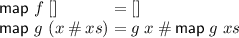Through elimination, this would turn into:

\begin{aligned} \mathsf {map} = \lambda \;&f \Rightarrow \big (\lambda \; [] \Rightarrow []\big ) \\ |\;&g \Rightarrow \big (\lambda \; x \mathbin {\#}xs \Rightarrow f\;x \mathbin {\#}\mathsf {map}\;f\;xs\big ) \end{aligned}

Even though the original equations were non-overlapping, we suddenly obtained an abstraction with two overlapping patterns. Slind observed a similar problem [38, Sect. 3.3.2] in his algorithm. Therefore, he only permits uniform equations, as defined by Wadler [36, Sect. 5.5]. Here, we can give a formal characterization of our requirements as a computable function on pairs of patterns:

This compatibility constraint ensures that any two overlapping patterns (of the same column) $$p_{i,k}$$ and $$p_{j,k}$$ are equal and are thus appropriately grouped together in the elimination procedure. We require all defining equations of a constant to be mutually compatible. Equations violating this constraint will be flagged during embedding (Sect. 3), whereas the pattern elimination algorithm always succeeds.

While this rules out some theoretically possible pattern combinations (e.g. the diagonal function [36, Sect. 5.5]), in practice, we have not found this to be a problem: All of the function definitions we have tried (Sect. 8) satisfied pattern compatibility (after automatic renaming of pattern variables). As a last resort, the user can manually instantiate function equations. Although this will always lead to a pattern compatible definition, it is not done automatically, due to the potential blow-up.

Discussion. Because this compilation phase is both non-trivial and has some minor restrictions on the set of function definitions that can be processed, we may provide an alternative implementation in the future. Instead of eliminating patterns from right to left, patterns may be grouped in tuples. The above example would be translated into:

\begin{aligned} \mathsf {map} = \lambda \;&(f, []) \Rightarrow [] \\ |\;&(f, x \mathbin {\#}xs) \Rightarrow f\;x \mathbin {\#}\mathsf {map}\;f\;xs \end{aligned}

We would then leave the compilation of patterns for the CakeML compiler, which has no pattern compatibility restriction.

The obvious disadvantage however is that this would require the knowledge of a tuple type in the term language which is otherwise unaware of concrete datatypes.

### 5.4 Sequentialization: Fromto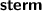The semantics of $$\mathsf {pterm}$$ and $$\mathsf {sterm}$$ differ only in rule Step and Beta. Figure 5 shows the modified rules. Instead of any matching clause, the first matching clause in a case abstraction is picked.

For the correctness proof, the order of clauses does not matter: we only need to prove that a step taken in the sequential semantics can be reproduced in the unordered semantics. As long as no rules are dropped, this is trivially true. For that reason, the compiler orders the clauses lexicographically. At the same time the rules are also converted from type $$(\mathsf {string}\times \mathsf {pterm})\;\mathsf {set}$$ to $$(\mathsf {string}\times \mathsf {sterm})\;\mathsf {list}$$. Below, $$\textit{rs}$$ will always denote a list of the latter type.

### 5.5 Big-Step Semantics forThis big-step semantics for $$\mathsf {sterm}$$ is not a compiler phase but moves towards the desired evaluation semantics. In this first step, we reuse the $$\mathsf {sterm}$$ type for evaluation results, instead of evaluating to the separate type $$\mathsf {value}$$. This allows us to ignore environment capture in closures for now.

All previous $$\longrightarrow$$ relations were parametrized by a rule set. Now the big-step predicate is of the form $$\textit{rs}, \sigma \vdash t \downarrow t'$$ where $$\sigma \, {:}{:}\, \mathsf {string}\rightharpoonup \mathsf {sterm}$$ is a variable environment.

This semantics also introduces the distinction between constructors and defined constants. If $$\mathsf {C}$$ is a constructor, the term $$\left\langle \mathsf {C}\;t_1\;\ldots \;t_n\right\rangle$$ is evaluated to $$\left\langle \mathsf {C}\;t'_1\;\ldots \;t'_n\right\rangle$$ where the $$t_i'$$ are the results of evaluating the $$t_i$$.

The full set of rules is shown in Fig. 6. They deserve a short explanation:

Const. :

Constants are retrieved from the rule set $$\textit{rs}$$.

Var. :

Variables are retrieved from the environment $$\sigma$$.

Abs. :

In order to achieve the intended invariant, abstractions are evaluated to their fully substituted form.

Comb. :

Function application $$t \;\\; u$$ first requires evaluation of t into an abstraction $$\varLambda \;\textit{cs}$$ and evaluation of u into an arbitrary term $$u'$$. Afterwards, we look for a clause matching $$u'$$ in $$\textit{cs}$$, which produces a local variable environment $$\sigma '$$, possibly overwriting existing variables in $$\sigma$$. Finally, we evaluate the right-hand side of the clause with the combined global and local variable environment.

Constr. :

For a constructor application $$\left\langle \mathsf {C}\;t_1\;\ldots \right\rangle$$, evaluate all $$t_i$$. The set constructors is an implicit parameter of the semantics.

### Lemma 1 (Closedness invariant)

If $$\sigma$$ contains only closed terms, $$\mathsf {frees}\;t \subseteq \mathsf {dom}\;\sigma$$ and $$\textit{rs}, \sigma \vdash t \downarrow t'$$, then $$t'$$ is closed.

Correctness of the big-step w.r.t. the small-step semantics is proved easily by induction on the former:

### Lemma 2

For any closed environment $$\sigma$$ satisfying $$\mathsf {frees}\;t \subseteq \mathsf {dom}\;\sigma$$,

\begin{aligned} \textit{rs}, \sigma \vdash t \downarrow u \rightarrow \textit{rs}\vdash {\mathsf {subst}\;\sigma \;t} \longrightarrow ^* u \end{aligned}

By setting $$\sigma = []$$, we obtain:

### Theorem 2 (Correctness)

$$\textit{rs}, [] \vdash t \downarrow u \wedge \mathsf {closed}\;t \rightarrow \textit{rs}\vdash t \longrightarrow ^* u$$

### 5.6 Evaluation Semantics: RefiningtoAt this point, we introduce the concept of values into the semantics, while still keeping the rule set (for constants) and the environment (for variables) separate. The evaluation rules are specified in Fig. 7 and represent a departure from the original rewriting semantics: a term does not evaluate to another term but to an object of a different type, a $$\mathsf {value}$$. We still use $$\downarrow$$ as notation, because big-step and evaluation semantics can be disambiguated by their types.

The evaluation model itself is fairly straightforward. As explained in Sect. 4.5, abstraction terms are evaluated to closures capturing the current variable environment. Note that at this point, recursive closures are not treated differently from non-recursive closures. In a later stage, when $$\textit{rs}$$ and $$\sigma$$ are merged, this distinction becomes relevant.

We will now explain each rule that has changed from the previous semantics:

Abs. :

Abstraction terms are evaluated to a closure capturing the current environment.

Comb. :

As before, in an application $$t\mathbin {\}u$$, t must evaluate to a closure $$\mathsf {Vabs}\;\textit{cs}\;\sigma '$$. The evaluation result of u is then matched against the clauses $$\textit{cs}$$, producing an environment $$\sigma ''$$. The right-hand side of the clause is then evaluated using $$\sigma '\mathbin {+\!\!+}\sigma ''$$; the original environment $$\sigma$$ is effectively discarded.

RecComb. :

Similar as above. Finding the matching clause is a two-step process: First, the appropriate clause list is selected by name of the currently active function. Then, matching is performed.

Constr. :

As before, for an n-ary application $$\left\langle \mathtt {C}\;t_1\;\ldots \right\rangle$$, where $$\mathsf {C}$$ is a data constructor, we evaluate all $$t_i$$. The result is a $$\mathsf {Vconstr}$$ value.

Conversion Betweenand. To establish a correspondence between evaluating a term to an $$\mathsf {sterm}$$ and to a $$\mathsf {value}$$, we apply the same trick as in Sect. 5.2. Instead of specifying a complicated relation, we translate $$\mathsf {value}$$ back to $$\mathsf {sterm}$$: simply apply the substitutions in the captured environments to the clauses.

The translation rules for $$\mathsf {Vabs}$$ and $$\mathsf {Vrecabs}$$ are kept similar to the Abs rule from the big-step semantics (Fig. 6). Roughly speaking, the big-step semantics always keeps terms fully substituted, whereas the evaluation semantics defers substitution.

Similarly to Sect. 5.2, we can also define a function $$\mathsf {sterm\_to\_value}\, {:}{:}\, \mathsf {sterm} \Rightarrow \mathsf {value}$$ and prove that one function is the inverse of the other.

Matching. The $$\mathsf {value}$$ type, instead of using binary function application as all other term types, uses n-ary constructor application. This introduces a conceptual mismatch between (binary) patterns and values. To make the proofs easier, we introduce an intermediate type of n-ary patterns. This intermediate type can be optimized away by fusion.

Correctness. The correctness proof requires a number of interesting lemmas.

### Lemma 3 (Substitution before evaluation)

Assuming that a term t can be evaluated to a value u given a closed environment $$\sigma$$, it can be evaluated to the same value after substitution with a sub-environment $$\sigma '$$. Formally: $$\textit{rs}, \sigma \vdash t \downarrow u \wedge \sigma ' \subseteq \sigma \rightarrow \textit{rs}, \sigma \vdash \mathsf {subst}\;\sigma '\;t \downarrow u$$

This justifies the “pre-substitution” exhibited by the Abs rule in the big-step semantics in contrast to the environment-capturing Abs rule in the evaluation semantics.

### Theorem 3 (Correctness)

Let $$\sigma$$ be a closed environment and t a term which only contains free variables in $$\mathsf {dom}\;\sigma$$. Then, an evaluation to a value $$\textit{rs}, \sigma \vdash t \downarrow v$$ can be reproduced in the big-step semantics as $$\textit{rs}', \mathsf {map}\;\mathsf {value\_to\_sterm}\;\sigma \vdash t \downarrow \mathsf {value\_to\_sterm}\;v$$, where $$\textit{rs}' = [( name , \mathsf {value\_to\_sterm}\; rhs ) \;|\; ( name , rhs ) \leftarrow \textit{rs}]$$.

Instantiating the Correctness Theorem. The correctness theorem states that, for any given evaluation of a term t with a given environment $$\textit{rs}, \sigma$$ containing $$\mathsf {value}$$s, we can reproduce that evaluation in the big-step semantics using a derived list of rules $$\textit{rs}'$$ and an environment $$\sigma '$$ containing $$\mathsf {sterm}$$s that are generated by the $$\mathsf {value\_to\_sterm}$$ function. But recall the diagram in Fig. 2. In our scenario, we start with a given rule set of $$\mathsf {sterm}$$s (that has been compiled from a rule set of $$\mathsf {term}$$s). Hence, the correctness theorem only deals with the opposite direction.

It remains to construct a suitable $$\textit{rs}$$ such that applying $$\mathsf {value\_to\_sterm}$$ to it yields the given $$\mathsf {sterm}$$ rule set. We can exploit the side condition (Sect. 5.1) that all bindings define functions, not constants:

### Definition 6 (Global clause set)

The mapping $$\mathsf {global\_css}\, {:}{:}\, \mathsf {string} \rightharpoonup ((\mathsf {term} \times \mathsf {sterm})\;\mathsf {list})$$ is obtained by stripping the $$\mathsf {Sabs}$$ constructors from all definitions and converting the resulting list to a mapping.

For each definition with name f we define a corresponding term $$v_f = \mathsf {Vrecabs}\;\mathsf {global\_css}\;f\;[]$$. In other words, each function is now represented by a recursive closure bundling all functions. Applying $$\mathsf {value\_to\_sterm}$$ to $$v_f$$ returns the original definition of f. Let $$\textit{rs}$$ denote the original $$\mathsf {sterm}$$ rule set and $$\textit{rs}_\text {v}$$ the environment mapping all f’s to the $$v_f$$’s.

The variable environments $$\sigma$$ and $$\sigma '$$ can safely be set to the empty mapping, because top-level terms are evaluated without any free variable bindings.

### Corollary 1 (Correctness)

$$\textit{rs}_\text {v}, [] \vdash t \downarrow v \rightarrow \textit{rs}, [] \vdash t \downarrow \mathsf {value\_to\_sterm}\;v$$

Note that this step was not part of the compiler (although $$\textit{rs}_\text {v}$$ is computable) but it is a refinement of the semantics to support a more modular correctness proof.

Example. Recall the $$\mathsf {odd}$$ and $$\mathsf {even}$$ example from Sect. 4.5. After compilation to $$\mathsf {sterm}$$, the rule set looks like this:

This can be easily transformed into the following global clause set:

Finally, $$\textit{rs}_\text {v}$$ is computed by creating a recursive closure for each function:

### 5.7 Evaluation with Recursive Closures

CakeML distinguishes between non-recursive and recursive closures . This distinction is also present in the $$\mathsf {value}$$ type. In this step, we will conflate variables with constants which necessitates a special treatment of recursive closures. Therefore we introduce a new predicate $$\sigma \vdash t \downarrow v$$ in Fig. 8 (in contrast to the previous $$\textit{rs}, \sigma \vdash t \downarrow v$$). We examine the rules one by one:

Const/Var. :

Constant definition and variable values are both retrieved from the same environment $$\sigma$$. We have opted to keep the distinction between constants and variables in the $$\mathsf {sterm}$$ type to avoid the introduction of another term type.

Abs. :

Identical to the previous evaluation semantics. Note that evaluation never creates recursive closures at run-time (only at compile-time, see Sect. 5.6). Anonymous functions, e.g. in the term $$\left\langle \mathsf {map}\;(\lambda x.\;x)\right\rangle$$, are evaluated to non-recursive closures.

Comb. :

Identical to the previous evaluation semantics.

RecComb. :

Almost identical to the evaluation semantics. Additionally, for each function $$( name , cs ) \in \textit{css}$$, a new recursive closure $$\mathsf {Vrecabs}\;\textit{css}\; name \;\sigma '$$ is created and inserted into the environment. This ensures that after the first call to a recursive function, the function itself is present in the environment to be called recursively, without having to introduce coinductive environments.

Constr. :

Identical to the evaluation semantics.

Conflating Constants and Variables. By merging the rule set $$\textit{rs}$$ with the variable environment $$\sigma$$, it becomes necessary to discuss possible clashes. Previously, the syntactic distinction between $$\mathsf {Svar}$$ and $$\mathsf {Sconst}$$ meant that $$\left\langle x\right\rangle$$ and $$\left\langle \mathsf {x}\right\rangle$$ are not ambiguous: all semantics up to the evaluation semantics clearly specify where to look for the substitute. This is not the case in functional languages where functions and variables are not distinguished syntactically.

Instead, we rely on the fact that the initial rule set only defines constants. All variables are introduced by matching before $$\beta$$-reduction (that is, in the Comb and RecComb rules). The Abs rule does not change the environment. Hence it suffices to assume that variables in patterns must not overlap with constant names (see Sect. 5.1).

Correspondence Relation. Both constant definitions and values of variables are recorded in a single environment $$\sigma$$. This also applies to the environment contained in a closure. The correspondence relation thus needs to take a different sets of bindings in closures into account.

Hence, we define a relation $$\approx _\text {v}$$ that is implicitly parametrized on the rule set $$\textit{rs}$$ and compares environments. We call it right-conflating, because in a correspondence $$v \approx _\text { v} u$$, any bound environment in u is thought to contain both variables and constants, whereas in v, any bound environment contains only variables.

### Definition 7 (Right-conflating correspondence)

We define $$\approx _\text {v}$$ coinductively as follows: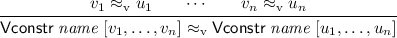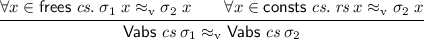Consequently, $$\approx _\text {v}$$ is not reflexive.

Correctness. The correctness lemma is straightforward to state:

### Theorem 4 (Correctness)

Let $$\sigma$$ be an environment, t be a closed term and v a value such that $$\sigma \vdash t \downarrow v$$. If for all constants x occurring in t, $$\textit{rs}\;x \approx _\text { v} \sigma \;x$$ holds, then there is an u such that $$\textit{rs}, [] \vdash t \downarrow u$$ and $$u \approx _\text { v} v$$.

As usual, the rather technical proof proceeds via induction over the semantics (Fig. 8). It is important to note that the global clause set construction (Sect. 5.6) satisfies the preconditions of this theorem:

### Lemma 4

If $$name$$ is the name of a constant in $$\textit{rs}$$, then

\begin{aligned} \mathsf {Vrecabs}\;\mathsf {global\_css}\; name \;[] \approx _\text { v} \mathsf {Vrecabs}\;\mathsf {global\_css}\; name \;[] \end{aligned}

Because $$\approx _\text {v}$$ is defined coinductively, the proof of this precondition proceeds by coinduction.

### 5.8 CakeML

CakeML is a verified implementation of a subset of Standard ML [24, 40]. It comprises a parser, type checker, formal semantics and backend for machine code. The semantics has been formalized in Lem , which allows export to Isabelle theories.

Our compiler targets CakeML’s abstract syntax tree. However, we do not make use of certain CakeML features; notably mutable cells, modules, and literals. We have derived a smaller, executable version of the original CakeML semantics, called CupCakeML, together with an equivalence proof. The correctness proof of the last compiler phase establishes a correspondence between CupCakeML and the final semantics of our compiler pipeline.

For the correctness proof of the CakeML compiler, its authors have extracted the Lem specification into HOL4 theories . In our work, we directly target CakeML abstract syntax trees (thereby bypassing the parser) and use its big-step semantics, which we have extracted into Isabelle.Footnote 2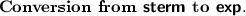After the series of translations described in the earlier sections, our terms are syntactically close to CakeML’s terms ($$\mathsf {Cake.exp}$$). The only remaining differences are outlined below:

• CakeML does not combine abstraction and pattern matching. For that reason, we have to translate $$\varLambda \;[p_1 \Rightarrow t_1, \ldots ]$$ into $$\varLambda x.\;\mathsf {\mathbf {case}}\;x\;\mathsf {\mathbf {of}}\;p_1 \Rightarrow t_1 \;|\; \ldots$$, where x is a fresh variable name. We reuse the $$\mathsf {fresh}$$ monad to obtain a bound variable name. Note that it is not necessary to thread through already created variable names, only existing names. The reason is simple: a generated variable is bound and then immediately used in the body. Shadowing it somewhere in the body is not problematic.

• CakeML has two distinct syntactic categories for identifiers (that can represent variables or functions) and data constructors. Our term types however have two distinct syntactic categories for constants (that can represent functions or data constructors) and variables. The necessary prerequisites to deal with this are already present in the ML-style evaluation semantics (Sect. 5.7) which conflates constants and variables, but has a dedicated Constr rule for data constructors.

Types. During embedding (Sect. 3), all type information is erased. Yet, CakeML performs some limited form of type checking at run-time: constructing and matching data must always be fully applied. That is, data constructors must always occur with all arguments supplied on right-hand and left-hand sides.

Fully applied constructors in terms can be easily guaranteed by simple pre-processing. For patterns however, this must be ensured throughout the compilation pipeline; it is (like other syntactic constraints) another side condition imposed on the rule set (Sect. 5.1).

The shape of datatypes and constructors is managed in CakeML’s environment. This particular piece of information is allowed to vary in closures, since ML supports local type definitions. Tracking this would greatly complicate our proofs. Hence, we fix a global set of constructors and enforce that all values use exactly that one.

Correspondence Relation. We define two different correspondence relations: One for values and one for expressions.

### Definition 8 (Expression correspondence)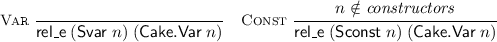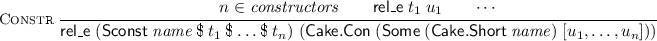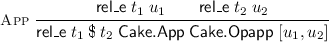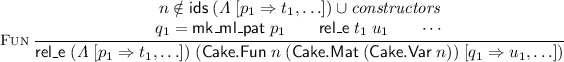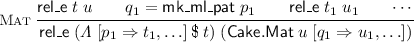We will explain each of the rules briefly here.

Var. :

Variables are directly related by identical name.

Const. :

As described earlier, constructors are treated specially in CakeML. In order to not confuse functions or variables with data constructors themselves, we require that the constant name is not a constructor.

Constr. :

Constructors are directly related by identical name, and recursively related arguments.

App. :

CakeML does not just support general function application but also unary and binary operators. In fact, function application is the binary operator $$\mathsf {Opapp}$$. We never generate other operators. Hence the correspondence is restricted to $$\mathsf {Opapp}$$.

Fun/Mat. :

Observe the symmetry between these two cases: In our term language, matching and abstraction are combined, which is not the case in CakeML. This means we relate a case abstraction to a CakeML function containing a match, and a case abstraction applied to a value to just a CakeML match.

There is no separate relation for patterns, because their translation is simple.

The value correspondence ($$\mathsf {rel\_v}$$) is structurally simpler. In the case of constructor values ($$\mathsf {Vconstr}$$ and $$\mathsf {Cake.Conv}$$), arguments are compared recursively. Closures and recursive closures are compared extensionally, i.e. only bindings that occur in the body are checked recursively for correspondence.

Correctness. We use the same trick as in Sect. 5.6 to obtain a suitable environment for CakeML evaluation based on the rule set $$\textit{rs}$$.

### Theorem 5 (Correctness)

If the compiled expression $$\mathsf {sterm\_to\_cake}\;t$$ terminates with a value u in the CakeML semantics, there is a value v such that $$\mathsf {rel\_v}\;v\;u$$ and $$\textit{rs}\vdash t \downarrow v$$.

## 6 Composition

The complete compiler pipeline consists of multiple phases. Correctness is justified for each phase between intermediate semantics and correspondence relations, most of which are rather technical. Whereas the compiler may be complex and impenetrable, the trustworthiness of the constructions hinges on the obviousness of those correspondence relations.

Fortunately, under the assumption that terms to be evaluated and the resulting values do not contain abstractions – or closures, respectively – all of the correspondence relations collapse to simple structural equality: two terms are related if and only if one can be converted to the other by consistent renaming of term constructors.

The actual compiler can be characterized with two functions. Firstly, the translation of $$\mathsf {term}$$ to $$\mathsf {Cake.exp}$$ is a simple composition of each term translation function:

Secondly, the function that translates function definitions by composing the phases as outlined in Fig. 2, including iterated application of pattern elimination:

Each function $$\mathsf {compile\_}$$* corresponds to one compiler phase; the remaining functions are trivial. This produces a CakeML top-level declaration. We prove that evaluating this declaration in the top-level semantics ($$\mathsf {evaluate\_prog}$$) results in an environment $$\mathsf {cake\_sem\_env}$$. But $$\mathsf {cake\_sem\_env}$$ can also be computed via another instance of the global clause set trick (Sect. 5.6).

Equipped with these functions, we can state the final correctness theorem:

This theorem directly relates the evaluation of a term t in the full CakeML (including mutability and exceptions) to the evaluation in the initial higher-order term rewriting semantics. The evaluation of t happens using the environment produced from the initial rule set. Hence, the theorem can be interpreted as the correctness of the pseudo-ML expression $$\mathsf {\mathbf {let\ rec}}\;\textit{rs}\;\mathsf {\mathbf {in}}\;t$$.

Observe that in the assumption, the conversion goes from our terms to CakeML expressions, whereas in the conclusion, the conversion goes the opposite direction.

## 7 Dictionary Construction

Isabelle’s type system supports type classes (or simply classes) [18, 44] whereas CakeML does not. In order to not complicate the correctness proofs, type classes are not supported by our embedded term language either. Instead, we eliminate classes and instances by a dictionary construction  before embedding into the term language. Haftmann and Nipkow give a pen-and-paper correctness proof of this construction [17, Sect. 4.1]. We augmented the dictionary construction with the generation of a certificate theorem that shows the equivalence of the two versions of a function, with type classes and with dictionaries. This section briefly explains our dictionary construction.

Figure 9 shows a simple example of a dictionary construction. Type variables may carry class constraints (e.g. $$\alpha \, {:}{:}\, \mathsf {add}$$). The basic idea is that classes become dictionaries containing the functions of that class; class instances become dictionary definitions. Dictionaries are realized as datatypes. Class constraints become additional dictionary parameters for that class. In the example, class $$\mathsf {add}$$ becomes $$\mathsf {dict\_add}$$; function f is translated into $$f'$$ which takes an additional parameter of type $$\mathsf {dict\_add}$$. In reality our tool does not produce the Isabelle source code shown in Fig. 9b but performs the constructions internally. The correctness lemma $$\mathsf {f'\_eq}$$ is proved automatically. Its precondition expresses that the dictionary must contain exactly the function(s) of class $$\mathsf {add}$$. For any monomorphic instance, the precondition can be proved outright based on the certificate theorems proved for each class instance as explained next.

Not shown in the example is the translation of class instances. The basic form of a class instance in Isabelle is $$\tau {:}{:} (c_1, \ldots , c_n)\;c$$ where $$\tau$$ is an n-ary type constructor. It corresponds to Haskell’s $$(c_1\;\alpha _1,\dots ,c_n\;\alpha _n) \Rightarrow c\;(\tau \;\alpha _1\dots \alpha _n)$$ and is translated into a function $$\mathsf {inst\_}c\mathsf {\_}\tau {:}{:} \alpha _1\;\mathsf {dict\_}c_1 \Rightarrow \cdots \Rightarrow \alpha _n\;\mathsf {dict\_}c_n \Rightarrow (\alpha _1,\dots ,\alpha _n)\;\tau \;\mathsf {dict\_}c$$ and the following certificate theorem is proved:

\begin{aligned} \mathsf {cert\_}c_1\; dict _1 \rightarrow \cdots \rightarrow \mathsf {cert\_}c_n\; dict _n \rightarrow \mathsf {cert\_}c\;(\mathsf {inst\_}c\mathsf {\_}\tau \; dict _1\;\ldots \; dict _n) \end{aligned}

For a more detailed explanation of how the dictionary construction works, we refer to the corresponding entry in the Archive of Formal Proofs .

## 8 Evaluation

We have tried out our compiler on examples from existing Isabelle formalizations. This includes an implementation of Huffman encoding, lists and sorting, string functions , and various data structures from Okasaki’s book , including binary search trees, pairing heaps, and leftist heaps. These definitions can be processed with slight modifications: functions need to be totalized (see the end of Sect. 3). However, parts of the tactics required for deep embedding proofs (Sect. 3) are too slow on some functions and hence still need to be optimized.

## 9 Conclusion

For this paper we have concentrated on the compiler from Isabelle/HOL to CakeML abstract syntax trees. Partial correctness is proved w.r.t. the big-step semantics of CakeML. In the next step we will link our work with the compiler from CakeML to machine code. Tan et al. [40, Sect. 10] prove a correctness theorem that relates their semantics with the execution of the compiled machine code. In that paper, they use a newer iteration of the CakeML semantics (functional big-step ) than we do here. Both semantics are still present in the CakeML source repository, together with an equivalence proof. Another important step consists of targeting CakeML’s native types, e.g. integer numbers and characters.

Evaluation of our compiled programs is already possible via Isabelle’s predicate compiler , which allows us to turn CakeML’s big-step semantics into an executable function. We have used this execution mechanism to establish for sample programs that they terminate successfully. We also plan to prove that our compiled programs terminate, i.e. total correctness.

The total size of this formalization, excluding theories extracted from Lem, is currently approximately 20000 lines of proof text (90 %) and ML code (10 %). The ML code itself produces relatively simple theorems, which means that there are less opportunities for it to go wrong. This constitutes an improvement over certifying approaches that prove complicated properties in ML.